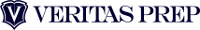# (GMAT 800) If n and a are positive integers, what is the units digit

If n and a are positive integers, what is the units digit of n^(4a+2) – n^(8a)?

(1) n = 3
(2) a is odd

A) Statement (1) ALONE is sufficient, but statement (2) alone is not sufficient to answer the question asked;
B)    Statement (2) ALONE is sufficient, but statement (1) alone is not sufficient to answer the question asked;
C)    BOTH statements (1) and (2) TOGETHER are sufficient to answer the question asked, but NEITHER statement ALONE is sufficient;
E)    Statements (1) and (2) TOGETHER are NOT sufficient to answer the question asked, and additional data are needed.

BONUS QUESTION: What actually is the units digit (assuming the answer is not E)?

One important thing to note about exponents is that, by definition, they indicate “repetitive multiplication” – the multiplication of the same number over and over again.  Accordingly, they lend themselves nicely to patterns, as when you perform the  same action over and over again you’ll tend to get similar results.  When you consider statement 1, that n = 3, look at how 3 multiplies to different exponents:

3^1 = 3
3^2 = 9
3^3 = 27
3^4  = 81

And to continue, we’ll just keep multiplying by 3; since the question is only asking about the units digit we’ll use the -> sign to indicate the units digit that the calculation will yield:

3^5 = 81 *3 -> 3
3^6 = 3*3 -> 9
3^7 = 9 * 3 -> 7
3^8 = 7 * 3 -> 1

If you notice, we’re in the midst of a repeating pattern: 3, 9, 7, 1, 3, 9, 7, 1…  The pattern dictates that it will recycle every fourth term, with each exponent-multiple-of-4 giving us a units digit of 1.  So we know that n^8a is going to end in 1.  And if you look closer, n^4a will also end in 1, and that first term from the question is n^(4a+2), meaning that each time we’ll go two iterations past the repeating 1.  Therefore, n^(4a+2) will always end in 9, and n^8a will always end in 1.  Accordingly, statement 1 is sufficient.

Statement 2, however, is not sufficient.  Without knowledge of n itself, we have no information about the base.  If n is 1, the units digit will always be 1; if n is 6, the units digit will always be 6.  Because the base of the exponent will dictate the possible values of the units digit, if we don’t have information about the base we won’t know the units digit, and therefore statement 2 is not sufficient.  Accordingly, the correct answer is A.

Solution: A

BONUS Solution:  Having read the above, you know that our units digits for n^(4a+2) – n^8a will be 9 – 1, so clearly the answer should be 8, right?  This is where the GMAT can become devilishly clever: the answer is not 8, and precious few examinees will answer this question correctly!  If you note the relative values of the exponents, 8a is always going to be bigger than 4a+2 (we are, after all, talking about a as a positive integer).  So we are not subtracting 9 – 1 with the left number being larger; instead, because the number on the right will be the larger number, our subtraction will take the form of 9 – 11, yielding a negative number that ends in 2.  The units digit of this subtraction will be 2, and should serve as a reminder that the GMAT reserves the right to test your level-of-consciousness: beware the often-overlooked number types negative, noninteger, and zero!Veritas Prep is the world’s largest privately-owned GMAT preparation and admissions consulting  provider, offering industry-leading programs to help applicants improve their test  scores and gain admission to the world’s best graduate schools. Founded in 2002 by graduates of the Yale School of Management, Veritas Prep is now live in more than 90 cities worldwide, as well as interactive online courses  available everywhere. Additionally, Veritas Prep offers industry-leading admissions consulting services for applicants seeking admission to the most competitive business schools, law schools, and medical schools in the world.1. Complete GMAT RC Questions in less than 1 minute and 50 seconds
3. Take Notes Effectively
4. Collect and Interpret Facts
5. Speed up Summary Creation
6. Remember Information
7. Question the Author

12. Learn to Answer GMAT organization of passage Question
13. Learn to identify the style/tone or attitude of the author

Mastering GMAT Critical ReasoningAfter you read F1GMAT’s Mastering GMAT Critical Reasoning Guide, you will learn:

How to overcome flawed thinking in GMAT Critical Reasoning?

How to spot Inconsistencies in Arguments

How to eliminate out of scope answer choices using Necessary and Sufficient Conditions

How to Paraphrase GMAT Critical Reasoning Question

How to Answer Assumption Question Type

How to Answer Conclusion Question Type

How to Answer Inference Question Type

How to Answer Strengthen Question Type

How to Answer Weaken Question Type

How to Answer bold-faced and Summary Question Types

How to Answer Parallel Reasoning Questions

How to Answer the Fill in the Blanks Question

Get F1GMAT's Newsletters (Best in the Industry)

• Ranking Analysis
• Post-MBA Salary Trends
• Post-MBA Job Function & Industry Analysis
• Post-MBA City Review
• MBA Application Essay Tips
• School Specific Essay Tips
• GMAT Preparation Tips